Chapter 1 The Nature of Science and Physics

# 3 1.2 Physical Quantities and Units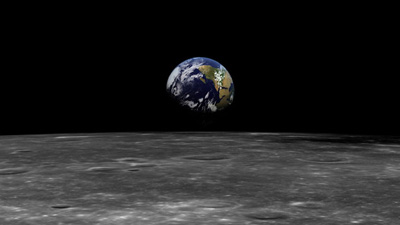The range of objects and phenomena studied in physics is immense. From the incredibly short lifetime of a nucleus to the age of the Earth, from the tiny sizes of sub-nuclear particles to the vast distance to the edges of the known universe, from the force exerted by a jumping flea to the force between Earth and the Sun, there are enough factors of 10 to challenge the imagination of even the most experienced scientist. Giving numerical values for physical quantities and equations for physical principles allows us to understand nature much more deeply than does qualitative description alone. To comprehend these vast ranges, we must also have accepted units in which to express them. And we shall find that (even in the potentially mundane discussion of meters, kilograms, and seconds) a profound simplicity of nature appears—all physical quantities can be expressed as combinations of only four fundamental physical quantities: length, mass, time, and electric current.

We define a physical quantity either by specifying how it is measured or by stating how it is calculated from other measurements. For example, we define distance and time by specifying methods for measuring them, whereas we define average speed by stating that it is calculated as distance traveled divided by time of travel.

Measurements of physical quantities are expressed in terms of units, which are standardized values. For example, the length of a race, which is a physical quantity, can be expressed in units of meters (for sprinters) or kilometers (for distance runners). Without standardized units, it would be extremely difficult for scientists to express and compare measured values in a meaningful way. (See Figure 2.)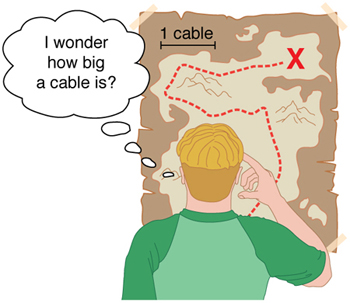There are two major systems of units used in the world: SI units (also known as the metric system) and English units (also known as the customary or imperial system). English units were historically used in nations once ruled by the British Empire and are still widely used in the United States. Virtually every other country in the world now uses SI units as the standard; the metric system is also the standard system agreed upon by scientists and mathematicians. The acronym “SI” is derived from the French Système International.

# SI Units: Fundamental and Derived Units

Table 1 gives the fundamental SI units that are used throughout this textbook. This text uses non-SI units in a few applications where they are in very common use, such as the measurement of blood pressure in millimeters of mercury (mm Hg). Whenever non-SI units are discussed, they will be tied to SI units through conversions.

Length Mass Time Electric Current
meter (m) kilogram (kg) second (s) ampere (A)
Table 1. Fundamental SI Units.

It is an intriguing fact that some physical quantities are more fundamental than others and that the most fundamental physical quantities can be defined only in terms of the procedure used to measure them. The units in which they are measured are thus called fundamental units. In this textbook, the fundamental physical quantities are taken to be length, mass, time, and electric current. (Note that electric current will not be introduced until much later in this text.) All other physical quantities, such as force and electric charge, can be expressed as algebraic combinations of length, mass, time, and current (for example, speed is length divided by time); these units are called derived units.

# Units of Time, Length, and Mass: The Second, Meter, and Kilogram

## The Second

The SI unit for time, the second (abbreviated s), has a long history. For many years it was defined as 1/86,400 of a mean solar day. More recently, a new standard was adopted to gain greater accuracy and to define the second in terms of a non-varying, or constant, physical phenomenon (because the solar day is getting longer due to very gradual slowing of the Earth’s rotation). Cesium atoms can be made to vibrate in a very steady way, and these vibrations can be readily observed and counted. In 1967 the second was redefined as the time required for 9,192,631,770 of these vibrations. (See Figure 3.) Accuracy in the fundamental units is essential, because all measurements are ultimately expressed in terms of fundamental units and can be no more accurate than are the fundamental units themselves.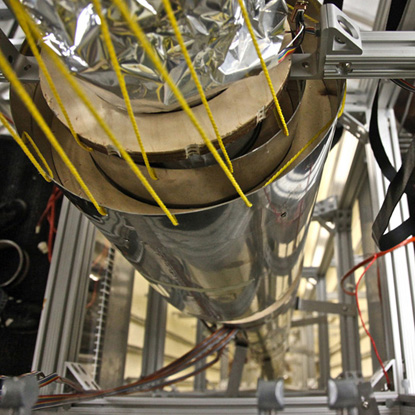## The Meter

The SI unit for length is the meter (abbreviated m); its definition has also changed over time to become more accurate and precise. The meter was first defined in 1791 as 1/10,000,000 of the distance from the equator to the North Pole. This measurement was improved in 1889 by redefining the meter to be the distance between two engraved lines on a platinum-iridium bar now kept near Paris. By 1960, it had become possible to define the meter even more accurately in terms of the wavelength of light, so it was again redefined as 1,650,763.73 wavelengths of orange light emitted by krypton atoms. In 1983, the meter was given its present definition (partly for greater accuracy) as the distance light travels in a vacuum in 1/299,792,458 of a second. (See Figure 4.) This change defines the speed of light to be exactly 299,792,458 meters per second. The length of the meter will change if the speed of light is someday measured with greater accuracy.

## The Kilogram

The SI unit for mass is the kilogram (abbreviated kg); it is defined to be the mass of a platinum-iridium cylinder kept with the old meter standard at the International Bureau of Weights and Measures near Paris. Exact replicas of the standard kilogram are also kept at the United States’ National Institute of Standards and Technology, or NIST, located in Gaithersburg, Maryland outside of Washington D.C., and at other locations around the world. The determination of all other masses can be ultimately traced to a comparison with the standard mass.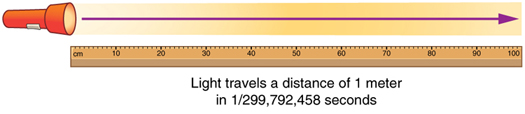Electric current and its accompanying unit, the ampere, will be introduced in when electricity and magnetism are covered. The initial modules in this textbook are concerned with mechanics, fluids, heat, and waves. In these subjects all pertinent physical quantities can be expressed in terms of the fundamental units of length, mass, and time.

# Metric Prefixes

SI units are part of the metric system. The metric system is convenient for scientific and engineering calculations because the units are categorized by factors of 10. Table 2 gives metric prefixes and symbols used to denote various factors of 10.

Metric systems have the advantage that conversions of units involve only powers of 10. There are 100 centimeters in a meter, 1000 meters in a kilometer, and so on. In nonmetric systems, such as the system of U.S. customary units, the relationships are not as simple—there are 12 inches in a foot, 5280 feet in a mile, and so on. Another advantage of the metric system is that the same unit can be used over extremely large ranges of values simply by using an appropriate metric prefix. For example, distances in meters are suitable in construction, while distances in kilometers are appropriate for air travel, and the tiny measure of nanometers are convenient in optical design. With the metric system there is no need to invent new units for particular applications.

The term order of magnitude refers to the scale of a value expressed in the metric system. Each power of 10 in the metric system represents a different order of magnitude. For example, 101, 102, 103, and so forth are all different orders of magnitude. All quantities that can be expressed as a product of a specific power of 10 are said to be of the same order of magnitude. For example, the number 800 can be written as 8 x 102, and the number 450 can be written as 4.5 x 102. Thus, the numbers 800 and 450 are of the same order of magnitude: 102. Order of magnitude can be thought of as a ballpark estimate for the scale of a value. The diameter of an atom is on the order of 10-9 m, while the diameter of the Sun is on the order of 109 m.

### THE QUEST FOR MICROSCOPIC STANDARDS FOR BASIC UNITS

The fundamental units described in this chapter are those that produce the greatest accuracy and precision in measurement. There is a sense among physicists that, because there is an underlying microscopic substructure to matter, it would be most satisfying to base our standards of measurement on microscopic objects and fundamental physical phenomena such as the speed of light. A microscopic standard has been accomplished for the standard of time, which is based on the oscillations of the cesium atom.

The standard for length was once based on the wavelength of light (a small-scale length) emitted by a certain type of atom, but it has been supplanted by the more precise measurement of the speed of light. If it becomes possible to measure the mass of atoms or a particular arrangement of atoms such as a silicon sphere to greater precision than the kilogram standard, it may become possible to base mass measurements on the small scale. There are also possibilities that electrical phenomena on the small scale may someday allow us to base a unit of charge on the charge of electrons and protons, but at present current and charge are related to large-scale currents and forces between wires.

Prefix Symbol Value 1 Example (some are approximate)
exa E 1018 exameter Em 1018 m distance light travels in a century
peta P 1015 petasecond Ps 1015 s 30 million years
tera T 1012 terawatt TW 1012 W powerful laser output
giga G 109 gigahertz GHz 109 Hz a microwave frequency
mega M 106 megacurie MCi 106 Ci high radioactivity
kilo k 103 kilometer km 103 m about 6/10 mile
hecto h 102 hectoliter hL 102 L 26 gallons
deka da 101 dekagram dag 101 g teaspoon of butter
100

(=1)

deci d 10-1 deciliter dL 10-1 L less than half a soda
centi c 10-2 centimeter cm 10-2 m fingertip thickness
milli m 10-3 millimeter mm 10-3 m flea at its shoulders
micro µ 10-6 micrometer µm 10-6 m detail in microscope
nano n 10-9 nanogram ng 10-9 g small speck of dust
pico p 10-12 picofarad pF 10-12 F small capacitor in radio
femto f 10-15 femtometer fm 10-15 m size of a proton
atto a 10-18 attosecond as 10-18 s time light crosses an atom
Table 2. Metric Prefixes for Powers of 10 and their Symbols.

# Known Ranges of Length, Mass, and Time

The vastness of the universe and the breadth over which physics applies are illustrated by the wide range of examples of known lengths, masses, and times in Table 3. Examination of this table will give you some feeling for the range of possible topics and numerical values. (See Figure 5 and Figure 6.)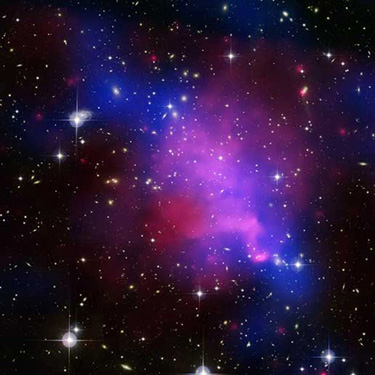# Unit Conversion and Dimensional Analysis

It is often necessary to convert from one type of unit to another. For example, if you are reading a European cookbook, some quantities may be expressed in units of liters and you need to convert them to cups. Or, perhaps you are reading walking directions from one location to another and you are interested in how many miles you will be walking. In this case, you will need to convert units of feet to miles.

Let us consider a simple example of how to convert units. Let us say that we want to convert 80 meters (m) to kilometers (km).

The first thing to do is to list the units that you have and the units that you want to convert to. In this case, we have units in meters and we want to convert to kilometers.

Next, we need to determine a conversion factor relating meters to kilometers. A conversion factor is a ratio expressing how many of one unit are equal to another unit. For example, there are 12 inches in 1 foot, 100 centimeters in 1 meter, 60 seconds in 1 minute, and so on. In this case, we know that there are 1,000 meters in 1 kilometer.

Now we can set up our unit conversion. We will write the units that we have and then multiply them by the conversion factor so that the units cancel out, as shown:

80 m = $\bf{\frac{1\textbf{ km}}{1000\;\rule[0.25ex]{0.75em}{0.1ex}\hspace{-0.75em}\textbf{m}}}$ = 0.080 km

Note that the unwanted m unit cancels, leaving only the desired km unit. You can use this method to convert between any types of unit.

Click Appendix C Useful Information for a more complete list of conversion factors.

Lengths in meters Masses in kilograms (more precise values in parentheses) Times in seconds (more precise values in parentheses)
10−18 Present experimental limit to smallest observable detail 10−30 Mass of an electron (9.11×10−31 kg) 10−23 Time for light to cross a proton
10−15 Diameter of a proton 10−27 Mass of a hydrogen atom (1.67×10−27 kg) 10−22 Mean life of an extremely unstable nucleus
10−14 Diameter of a uranium nucleus 10−15 Mass of a bacterium 10−15 Time for one oscillation of visible light
10−10 Diameter of a hydrogen atom 10−5 Mass of a mosquito 10−13 Time for one vibration of an atom in a solid
10−8 Thickness of membranes in cells of living organisms 10−2 Mass of a hummingbird 10−8 Time for one oscillation of an FM radio wave
10−6 Wavelength of visible light 1 Mass of a liter of water (about a quart) 10−3 Duration of a nerve impulse
10−3 Size of a grain of sand 102 Mass of a person 1 Time for one heartbeat
1 Height of a 4-year-old child 103 Mass of a car 105 One day (8.64×104 s)
102 Length of a football field 108 Mass of a large ship 107 One year (y) (3.16×107 s)
104 Greatest ocean depth 1012 Mass of a large iceberg 109 About half the life expectancy of a human
107 Diameter of the Earth 1015 Mass of the nucleus of a comet 1011 Recorded history
1011 Distance from the Earth to the Sun 1023 Mass of the Moon (7.35×1022 kg) 1017 Age of the Earth
1016 Distance traveled by light in 1 year (a light year) 1025 Mass of the Earth (5.97×1024 kg) 1018 Age of the universe
1021 Diameter of the Milky Way galaxy 1030 Mass of the Sun (1.99×1030 kg)
1022 Distance from the Earth to the nearest large galaxy (Andromeda) 1042 Mass of the Milky Way galaxy (current upper limit)
1026 Distance from the Earth to the edges of the known universe 1053 Mass of the known universe (current upper limit)
Table 3. Approximate Values of Length, Mass, and Time.

### Example 1: Unit Conversions: A Short Drive Home

Suppose that you drive the 10.0 km from your university to home in 20.0 min. Calculate your average speed (a) in kilometers per hour (km/h) and (b) in meters per second (m/s). (Note: Average speed is distance traveled divided by time of travel.)

Strategy

First we calculate the average speed using the given units. Then we can get the average speed into the desired units by picking the correct conversion factor and multiplying by it. The correct conversion factor is the one that cancels the unwanted unit and leaves the desired unit in its place.

Solution for (a)

(1) Calculate average speed. Average speed is distance traveled divided by time of travel. (Take this definition as a given for now—average speed and other motion concepts will be covered in a later module.) In equation form,

average speed = $\bf{\frac {\textbf{distance}}{\textbf{time}}}$

(2) Substitute the given values for distance and time.

average speed = $\bf{\frac {10.0\textbf{ km}} {20.0\textbf{ min}}}$ = 0.500 km/min

(3) Convert km/min to km/h: multiply by the conversion factor that will cancel minutes and leave hours. That conversion factor is 60 min/h. Thus,

average speed = 0.500 km/min x $\bf{\frac{60\textbf{ min}}{1\textbf{ h}}}$ = 30.0 km/h

Discussion for (a)

To check your answer, consider the following:

(1) Be sure that you have properly cancelled the units in the unit conversion. If you have written the unit conversion factor upside down, the units will not cancel properly in the equation. If you accidentally get the ratio upside down, then the units will not cancel; rather, they will give you the wrong units as follows:

$\bf{\frac{km}{min}}$ $\bf{\times}$ $\bf{\frac{1\textbf{ hr}}{60\textbf{ min}}}$ $\bf{=}$ $\bf{\frac{1\textbf{ km}\cdot\textbf{hr}}{60\textbf{ min}^2}}$,

which are obviously not the desired units of km/h.

(2) Check that the units of the final answer are the desired units. The problem asked us to solve for average speed in units of km/h and we have indeed obtained these units.

(3) Check the significant figures. Because each of the values given in the problem has three significant figures, the answer should also have three significant figures. The answer$30.0 \frac {km} {hr}$ does indeed have three significant figures, so this is appropriate. Note that the significant figures in the conversion factor are not relevant because an hour is defined to be 60 minutes, so the precision of the conversion factor is perfect.

(4) Next, check whether the answer is reasonable. Let us consider some information from the problem—if you travel 10 km in a third of an hour (20 min), you would travel three times that far in an hour. The answer does seem reasonable.

Solution for (b)

There are several ways to convert the average speed into meters per second.

(1) Start with the answer to (a) and convert km/h to m/s. Two conversion factors are needed—one to convert hours to seconds, and another to convert kilometers to meters.

(2) Multiplying by these yields

average speed = $\bf{\frac{km}{h}}$ $\bf{\times}$ $\bf{\frac{1\textbf{ h}}{3,600\textbf{ s}}}$$\bf{\times}$$\bf{\frac{1,000\textbf{ m}} {1\textbf{ km}}}$,
average speed = 8.33 m/s

Discussion for (b)

If we had started with 0.500 km/min, we would have needed different conversion factors, but the answer would have been the same: 8.33 m/s.

You may have noted that the answers in the worked example just covered were given to three digits. Why? When do you need to be concerned about the number of digits in something you calculate? Why not write down all the digits your calculator produces? The module Chapter 1.3 Accuracy, Precision, and Significant Figures will help you answer these questions.

### NONSTANDARD UNITS

While there are numerous types of units that we are all familiar with, there are others that are much more obscure. For example, a firkin is a unit of volume that was once used to measure beer. One firkin equals about 34 liters. To learn more about nonstandard units, use a dictionary or encyclopedia to research different “weights and measures.” Take note of any unusual units, such as a barleycorn, that are not listed in the text. Think about how the unit is defined and state its relationship to SI units.

### Check Your Understanding 1

1: Some hummingbirds beat their wings more than 50 times per second. A scientist is measuring the time it takes for a hummingbird to beat its wings once. Which fundamental unit should the scientist use to describe the measurement? Which factor of 10 is the scientist likely to use to describe the motion precisely? Identify the metric prefix that corresponds to this factor of 10.

### Check Your Understanding 2

1: One cubic centimeter is equal to one milliliter. What does this tell you about the different units in the SI metric system?

# Summary

• Physical quantities are a characteristic or property of an object that can be measured or calculated from other measurements.
• Units are standards for expressing and comparing the measurement of physical quantities. All units can be expressed as combinations of four fundamental units.
• The four fundamental units we will use in this text are the meter (for length), the kilogram (for mass), the second (for time), and the ampere (for electric current). These units are part of the metric system, which uses powers of 10 to relate quantities over the vast ranges encountered in nature.
• The four fundamental units are abbreviated as follows: meter, m; kilogram, kg; second, s; and ampere, A. The metric system also uses a standard set of prefixes to denote each order of magnitude greater than or lesser than the fundamental unit itself.
• Unit conversions involve changing a value expressed in one type of unit to another type of unit. This is done by using conversion factors, which are ratios relating equal quantities of different units.

### Conceptual Questions

1: Identify some advantages of metric units.

### Problems & Exercises

1: The speed limit on some interstate highways is roughly 100 km/h. (a) What is this in meters per second? (b) How many miles per hour is this?

2: A car is traveling at a speed of 33 m/s. (a) What is its speed in kilometers per hour? (b) Is it exceeding the 90 km/h speed limit?

3: Show that 1.0 m/s = 3.6 km/h. Hint: Show the explicit steps involved in converting 1.0 m/s = 3.6 km/h.

4: American football is played on a 100-yd-long field, excluding the end zones. How long is the field in meters? (Assume that 1 meter equals 3.281 feet.)

5: Soccer fields vary in size. A large soccer field is 115 m long and 85 m wide. What are its dimensions in feet and inches? (Assume that 1 meter equals 3.281 feet.)

6: What is the height in meters of a person who is 6 ft 1.0 in. tall? (Assume that 1 meter equals 39.37 in.)

7: Mount Everest, at 29,028 feet, is the tallest mountain on the Earth. What is its height in kilometers? (Assume that 1 kilometer equals 3,281 feet.)

8: The speed of sound is measured to be 342 m/s on a certain day. What is this in km/h?

9: Tectonic plates are large segments of the Earth’s crust that move slowly. Suppose that one such plate has an average speed of 4.0 cm/year. (a) What distance does it move in 1 s at this speed? (b) What is its speed in kilometers per million years?

10: (a) Refer to Table 3 to determine the average distance between the Earth and the Sun. Then calculate the average speed of the Earth in its orbit in kilometers per second. (b) What is this in meters per second?

# Glossary

physical quantity
a characteristic or property of an object that can be measure or calculated from other measurements
units
a standard used for expressing and comparing measurements
SI units
the international system of units that scientist in most countries have agreed to use; includes units such as meters, liters, and grams
English units
system of measurement used in the United States; includes units of measurement such as feet, gallons, and pounds
fundamental units
units that can only be expressed relative to the procedure used to measure them
derived units
units that can be calculated using algebraic combinations of the fundamental units
second
the SI unit for time, abbreviated (s)
meter
the SI unit for length, abbreviated (m)
kilogram
the SI unit for mass, abbreviated (kg)
metric system
a system in which values can be calculated in factors of 10
order of magnitude
refers to the size of a quantity as it related to a power of 10
conversion factor
a ratio expression how many of one unit are equal to another unit

### Solutions

Check Your Understanding 1

1: The scientist will measure the time between each movement using the fundamental unit of seconds. Because the wings beat so fast, the scientist will probably need to measure in milliseconds, or 10-3 seconds. (50 beats per second corresponds to 20 milliseconds per beat.)

Check Your Understanding 2

1: The fundamental unit of length (meter) is probably used to create the derived unit of volume (liter). The measure of a milliliter is dependent on the measure of a centimeter.

Problems & Exercises

1a. 27.8 m/s

1b. 62.1 mph

3.  $\bf{\frac{1.0\textbf{ m}}{s}=\frac{1.0\textbf{ m}}{s}\times\frac{3600\textbf{ s}}{1\textbf{ hr}}\times\frac{1\textbf{ km}}{1000\textbf{ m}}}$ = 3.6 km/h

5.  length: 377  ft;  4.53 x 103 in. / width: 280 ft;  3.3 x 103 in.

7. 8.847 km

9a. 1.3 x 10-9 m

9b. 40 km/My

## LicenseCollege Physics: OpenStax by OpenStax is licensed under a Creative Commons Attribution 4.0 International License, except where otherwise noted.